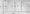# Electronic Devices and Circuits Feb-2011

Unit-I

1.a) Sketch the energy-band picture for

i)    an intrinsic,

ii)   an n-type, and

iii)  a p-type semiconductor. Indicate the position of the fermi, the donor, and the acceptor levels.

b) A semiconductor is doped with both donors and acceptors of concentrations Nd, and Na, respectively. Write the equation or equations from which to determine the electron and hole concentration (n and p)

OR

a)  Describe the Hall effect. What properties of a semiconductor are determined from a Hal 1 efleet experiment?

b)  W;rite the equation of continuity for holes. Explain the physical meaning of each term in the equation.

Unit – II

2. a) Draw the piecewise linear volt-ampere characteristic of a p-n diode. What are the circuit model for the ON state and the OFF state.

b) Determine V0,1,, ID,, and ID2 for the parallel diode configuration of fig. 1 (8) 0.33KnOR

a)     Draw the UJT static emitter characteristic curve and UJT equivalent circuit.

b) Determine the current I for the network of Fig.2

3. a) Write the Ebers and Moll equations and sketch the circuit model which satisfies these equations.

b) Determine the following for the fixed-bias configuration of fig.3OR

a)  List the three sources of instability of collector current and define the three stability factors.

b)     Find hre in terms of the CB h-parameters.

Unit-IV

4.  a) Show the small-signal model of an FET at low frequencies and at high frequencies.

b) Sketch the circuit of a CS amplifier. Derive the expression for the voltage gain at low frequencies.

OR

a)     Sketch the cross section of a P-channel enhancement MOSFET.

b)  Draw the E – MOSFET voltage divider configuration and their AC equivalent network.

c)   D erive the relation for g for enhancement type MOSFETs.

Unit -V

5. a) Describe the Miller’s theorem.

b) Calculate the voltage gain, output voltage, input impedance, and output impedance for the cascade BJT amplifier of fig.4. Calculate the output voltage resulting if a 10 KQ load is connected to the output.OR

a)  Draw a Darlington emitter follower and explain why the input impedance is higher than that of a single-stage emitter follower.

b)  Draw the circuit of an emitter coupled differential amplifier. Also draw the transfer characteristics of a diiYerential amplifier.